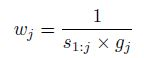## 5 mar. 2017

### Wheigthed Average (LOCAL)

We have seen in the post  LOCAL optimization  how, when giving a prediction, LOCAL uses all the PLS terms range we have fixed in the options Min to Max number of terms, and the result is a weighted average of all the results predictions of all the models. So to choose the right range is important to get more accurate predictions.
Looking in the Resemble R package documentation you can see some explanations about how the calculations are made:

"Weighted average pls ("wapls1"): It uses multiple models generated by multiple pls components (i.e. between a minimum and a maximum number of pls components). At each local partition the final predicted value is a weighted average of all the predicted values generated by the multiple pls models. The weight for each component is calculated as follows":"where s1:j  is the root mean square of the spectral residuals of the unknown (or target) sample when a total of j pls components are used and gj is the root mean square of the regression coefficients corresponding to the jth pls component (see Shenk et al., 1997 for more details).
"wapls1" is not compatible with valMethod = "loc_crossval" since the weights are computed based on the sample to be predicted at each local iteration.
by the multiple pls models".